Like   Tweet   in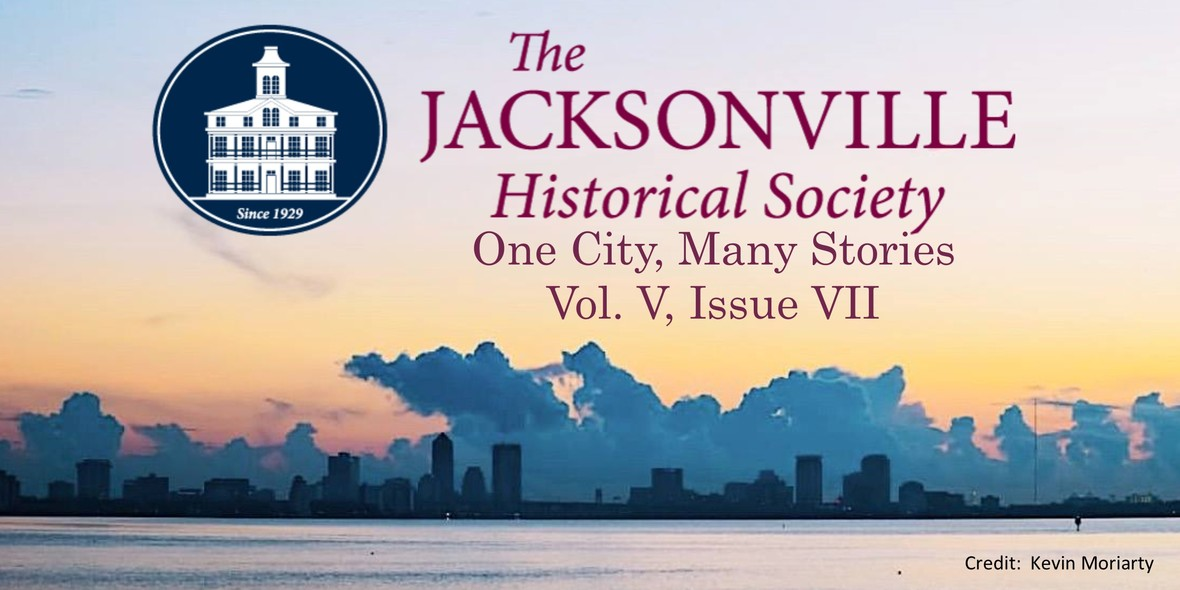# Jacksonville History Matters: NEWS & EVENTS

 table div table+table+table+table div table{width:100%;padding:0}table div table+table+table+table div table img{width:96.23%;padding:0;float:none}table div table+table+table+table div table td{width:100%;padding:0 1.88% 18px}/* styles */## Speaking of Jacksonville

 table.module-5{width:99.81%;padding:0}table div table+table+table+table+table+table div table{width:99.81%;float:none;margin-left:auto;margin-right:auto;padding:0}table div table+table+table+table+table+table div table a{border:0 none;text-decoration:none}table div table+table+table+table+table+table div table img{width:100%!important;border:0 none;text-decoration:none}table div table+table+table+table+table+table div table td{width:100%;padding:0}/* styles */
 table div table+table+table+table+table+table+table+table+table div table{width:100%;padding:0}table div table+table+table+table+table+table+table+table+table div table img{width:96.23%;padding:0;float:none}table div table+table+table+table+table+table+table+table+table div table td{width:100%;padding:0 1.88% 18px}/* styles */table div table+table+table+table+table+table+table+table+table+table div table,table.module-9{width:48.68%;float:right;padding:0}table div table+table+table+table+table+table+table+table+table+table div table a{border:0 none;text-decoration:none}table div table+table+table+table+table+table+table+table+table+table div table img{width:100%!important;border:0 none;text-decoration:none}table div table+table+table+table+table+table+table+table+table+table div table td{width:100%;padding:0 0 20px 20px}/* styles */ Have you ever heard Jacksonville described as “The Hartford of the South”? The nickname was appropriate, as during the twentieth century our city became home to more than a dozen insurance companies, offering life, property & casualty, and title insurance. Among the first was the Afro-American Life Insurance Company, chartered here in Jacksonville on March 1, 1901 – two months before the Great Fire. From that beginning, the insurance industry came to be one of the dominant factors in Jacksonville’s economy and civic life. No one is more capable to share that story than Jacob F. Bryan, IV, formerly President of Jacksonville’s home-grown Independent Insurance Group. Mr. Bryan will speak at Old St. Andrew’s on the evening of Thursday, September 5, 2019. Please make a reservation at the link below if you plan to join us. JHS Members receive free admission to this event. Non-members \$10.
 table div table+table+table+table+table+table+table+table+table+table+table+table div table{width:100%;padding:0}table div table+table+table+table+table+table+table+table+table+table+table+table div table img{width:96.23%;padding:0;float:none}table div table+table+table+table+table+table+table+table+table+table+table+table div table td{width:100%;padding:0 1.88% 18px}/* styles *//* styles */ The motto of the Jacksonville Historical Society, “One City, Many Stories,” expresses our mission and role across the community. We want to have you with us at these and future programs, as we learn about the people and events that have shaped Jacksonville, past, present and future. And, we want to know how we can help share your stories! Alan Bliss Executive Director
 table div table+table+table+table+table+table+table+table+table+table+table+table+table+table+table div table{width:100%;padding:0}table div table+table+table+table+table+table+table+table+table+table+table+table+table+table+table div table img{width:96.23%;padding:0;float:none}table div table+table+table+table+table+table+table+table+table+table+table+table+table+table+table div table td{width:100%;padding:0 1.88% 18px}/* styles */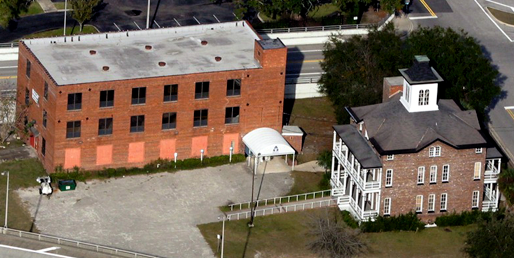Florida Casket Factory and Old St. Luke's Hospital

Do you know that the Jacksonville Historical Society owns a casket factory? It has been decades since the Florida Casket Company operated from its three-story brick headquarters at 318 Palmetto Street. Today, the building is a part of the JHS’s historic campus, right next door to the Old St. Luke’s Hospital, and is overdue for significant renovation. Meeting that need has long been one of the JHS’s most urgent goals, and a campaign is now underway for capital contributions to activate the second floor. Jacksonville deserves a historical society capable of employing the best practices and professional standards in archival stewardship. When complete, the JHS will gain 4500 square feet of safe, up-to-date, climate-controlled space for the processing and preservation of sensitive archives, including tens of thousands of photos and photo negatives. This has been part of our strategic plan since 2016, and we are excited to have reached this stage in achieving it.

Thanks to a volunteer team of design and construction professionals from the Haskell Company, a feasibility report and project budget are now in hand. Will you invest in the future of the Jacksonville Historical Society by contributing to this campaign? For more information, please contact Executive Director Alan Bliss, at 904-665-0064, or alan.bliss@jaxhistory.org

 table div table+table+table+table+table+table+table+table+table+table+table+table+table+table+table+table+table+table div table{width:100%;padding:0}table div table+table+table+table+table+table+table+table+table+table+table+table+table+table+table+table+table+table div table img{width:96.23%;padding:0;float:none}table div table+table+table+table+table+table+table+table+table+table+table+table+table+table+table+table+table+table div table td{width:100%;padding:0 1.88% 18px}/* styles */## Fun with the Jax Red Caps!

 /* styles */ On July 13, we enjoyed an action-packed night in collaboration with the Durkeeville Historical Society. We had the pleasure of listening to Mr. Lloyd Washington, President of the Durkeeville Historical Society and Otis "Crazy Boy" Williams, former baseball player of the Indianapolis Clowns and relief pitcher for Satchel Paige. After the presentation, we enjoyed an exciting Jumbo Shrimp game in honor of the Jax Red Caps!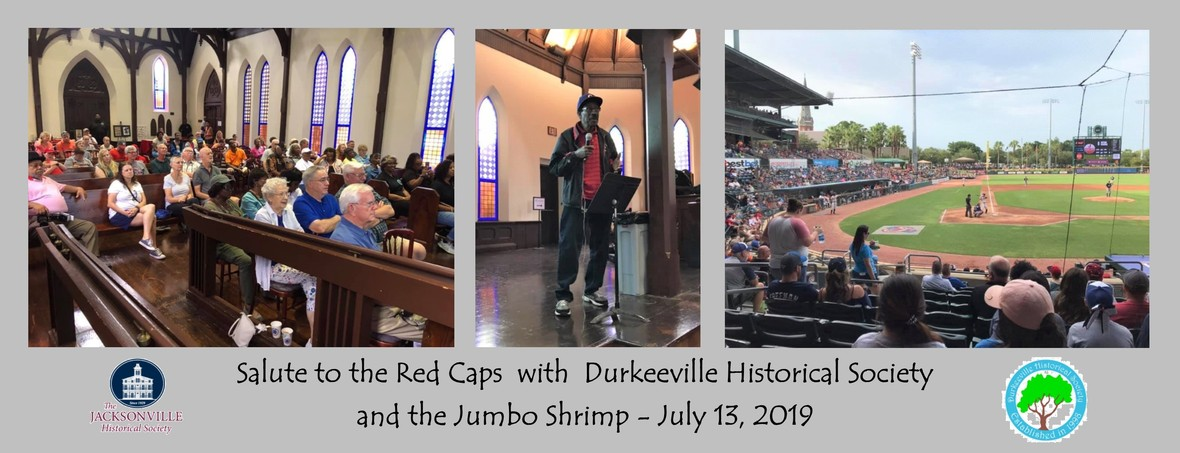/* styles */ The Jax Red Caps had a relatively short tenure in the Negro American League. They played in Jacksonville in the 1938, 1941, and 1942 seasons. Although their time in the Negro American League was short, the team is historically significant to Jacksonville. The Red Caps home field was Barr’s Field in Jacksonville, now known as J. P. Small Memorial Park. The ballpark, located in the Durkeeville neighborhood, is the site of much history for both black and white baseball teams and was accepted to the National Register for Historic Places in 2013. Thank you Durkeeville folks for sharing your talents and your legacy with all of us!
 table div table+table+table+table+table+table+table+table+table+table+table+table+table+table+table+table+table+table+table+table+table+table+table div table{width:100%;padding:0}table div table+table+table+table+table+table+table+table+table+table+table+table+table+table+table+table+table+table+table+table+table+table+table div table img{width:96.23%;padding:0;float:none}table div table+table+table+table+table+table+table+table+table+table+table+table+table+table+table+table+table+table+table+table+table+table+table div table td{width:100%;padding:0 1.88% 18px}/* styles */## Community and Collaboration

 /* styles */ This July I had the extreme pleasure of participating in a two day conference at the University of Florida entitled Digital Collaborations on Black History in Florida. The event was held at the George A. Smathers Libraries with approximately 80 individuals in attendance from archives, libraries and cultural institutions around the state. The conversations were stimulating and the workshops were eye opening. I left with a renewed sense of purpose, along with many strategies I hope to implement at the Jacksonville Historical Society.
 table.module-25{width:99.62%;padding:0}table div table+table+table+table+table+table+table+table+table+table+table+table+table+table+table+table+table+table+table+table+table+table+table+table+table+table div table{width:99.62%;float:none;margin-left:auto;margin-right:auto;padding:0}table div table+table+table+table+table+table+table+table+table+table+table+table+table+table+table+table+table+table+table+table+table+table+table+table+table+table div table a{border:0 none;text-decoration:none}table div table+table+table+table+table+table+table+table+table+table+table+table+table+table+table+table+table+table+table+table+table+table+table+table+table+table div table img{width:100%!important;border:0 none;text-decoration:none}table div table+table+table+table+table+table+table+table+table+table+table+table+table+table+table+table+table+table+table+table+table+table+table+table+table+table div table td{width:100%;padding:0}/* styles */
 /* styles */ My biggest takeaway was the idea that communities build collections. If you think about it, they are the source and we are the resource. The stories of the African American community are not my stories, but then again, none of the stories preserved at the JHS are my stories. They are your stories, and it’s my duty to ensure that they are properly preserved and made accessible to the community as a whole for generations to come. We’re grateful to the many diverse communities of Jacksonville for allowing us to tell your stories for more than 90 years, and we look forward to continuing that legacy of trust. On the subject of Black history, this summer marks the 100th Anniversary of the Red Summer of 1919, a turbulent time in America marked with increased racial violence and rioting throughout the country following the end of World War I. Keep an eye out for a lengthier piece on the Red Summer and its affects on Jacksonville in next month's newsletter. by Mitch Hemann Archivist
 table div table+table+table+table+table+table+table+table+table+table+table+table+table+table+table+table+table+table+table+table+table+table+table+table+table+table+table+table div table{width:100%;padding:0}table div table+table+table+table+table+table+table+table+table+table+table+table+table+table+table+table+table+table+table+table+table+table+table+table+table+table+table+table div table img{width:96.23%;padding:0;float:none}table div table+table+table+table+table+table+table+table+table+table+table+table+table+table+table+table+table+table+table+table+table+table+table+table+table+table+table+table div table td{width:100%;padding:0 1.88% 18px}/* styles */table.module-29{width:75.47%;padding:0}table div table+table+table+table+table+table+table+table+table+table+table+table+table+table+table+table+table+table+table+table+table+table+table+table+table+table+table+table+table+table div table{width:75.47%;float:none;margin-left:auto;margin-right:auto;padding:0}table div table+table+table+table+table+table+table+table+table+table+table+table+table+table+table+table+table+table+table+table+table+table+table+table+table+table+table+table+table+table div table a{border:0 none;text-decoration:none}table div table+table+table+table+table+table+table+table+table+table+table+table+table+table+table+table+table+table+table+table+table+table+table+table+table+table+table+table+table+table div table img{width:100%!important;border:0 none;text-decoration:none}table div table+table+table+table+table+table+table+table+table+table+table+table+table+table+table+table+table+table+table+table+table+table+table+table+table+table+table+table+table+table div table td{width:100%;padding:0}/* styles */
 table div table+table+table+table+table+table+table+table+table+table+table+table+table+table+table+table+table+table+table+table+table+table+table+table+table+table+table+table+table+table+table+table+table+table div table{width:100%;padding:0}table div table+table+table+table+table+table+table+table+table+table+table+table+table+table+table+table+table+table+table+table+table+table+table+table+table+table+table+table+table+table+table+table+table+table div table img{width:96.23%;padding:0;float:none}table div table+table+table+table+table+table+table+table+table+table+table+table+table+table+table+table+table+table+table+table+table+table+table+table+table+table+table+table+table+table+table+table+table+table div table td{width:100%;padding:0 1.88% 18px}/* styles */table.module-34{width:75.47%;padding:0}table div table+table+table+table+table+table+table+table+table+table+table+table+table+table+table+table+table+table+table+table+table+table+table+table+table+table+table+table+table+table+table+table+table+table+table div table{width:75.47%;float:none;margin-left:auto;margin-right:auto;padding:0}table div table+table+table+table+table+table+table+table+table+table+table+table+table+table+table+table+table+table+table+table+table+table+table+table+table+table+table+table+table+table+table+table+table+table+table div table a{border:0 none;text-decoration:none}table div table+table+table+table+table+table+table+table+table+table+table+table+table+table+table+table+table+table+table+table+table+table+table+table+table+table+table+table+table+table+table+table+table+table+table div table img{width:100%!important;border:0 none;text-decoration:none}table div table+table+table+table+table+table+table+table+table+table+table+table+table+table+table+table+table+table+table+table+table+table+table+table+table+table+table+table+table+table+table+table+table+table+table div table td{width:100%;padding:0}/* styles */

## Holiday Party Reservations at Old St. Andrews!

 /* styles */ Shake off the hassles and work of holiday party planning. Old St. Andrews and the Gingerbread Extravaganza are available for evening holiday parties this December! Impress your friends, clients or staff by hosting your holiday party in the ambiance of Old St. Andrews decorated in Gingerbread Extravaganza splendor. Our Rental Coordinators have catering resources ready to provide a turnkey event for you! For more information, contact our Rental Coordinators: Jade Stanley: 904-878-8122 Rob Jackson: 904-878-2241 Email: Events@JaxHistory.org
 table div table+table+table+table+table+table+table+table+table+table+table+table+table+table+table+table+table+table+table+table+table+table+table+table+table+table+table+table+table+table+table+table+table+table+table+table+table+table div table{width:100%;padding:0}table div table+table+table+table+table+table+table+table+table+table+table+table+table+table+table+table+table+table+table+table+table+table+table+table+table+table+table+table+table+table+table+table+table+table+table+table+table+table div table img{width:96.23%;padding:0;float:none}table div table+table+table+table+table+table+table+table+table+table+table+table+table+table+table+table+table+table+table+table+table+table+table+table+table+table+table+table+table+table+table+table+table+table+table+table+table+table div table td{width:100%;padding:0 1.88% 18px}/* styles */## Upcoming Programs and Events

 table.module-39{width:75.47%;padding:0}table div table+table+table+table+table+table+table+table+table+table+table+table+table+table+table+table+table+table+table+table+table+table+table+table+table+table+table+table+table+table+table+table+table+table+table+table+table+table+table+table div table{width:75.47%;float:none;margin-left:auto;margin-right:auto;padding:0}table div table+table+table+table+table+table+table+table+table+table+table+table+table+table+table+table+table+table+table+table+table+table+table+table+table+table+table+table+table+table+table+table+table+table+table+table+table+table+table+table div table a{border:0 none;text-decoration:none}table div table+table+table+table+table+table+table+table+table+table+table+table+table+table+table+table+table+table+table+table+table+table+table+table+table+table+table+table+table+table+table+table+table+table+table+table+table+table+table+table div table img{width:100%!important;border:0 none;text-decoration:none}table div table+table+table+table+table+table+table+table+table+table+table+table+table+table+table+table+table+table+table+table+table+table+table+table+table+table+table+table+table+table+table+table+table+table+table+table+table+table+table+table div table td{width:100%;padding:0}/* styles */
 table div table+table+table+table+table+table+table+table+table+table+table+table+table+table+table+table+table+table+table+table+table+table+table+table+table+table+table+table+table+table+table+table+table+table+table+table+table+table+table+table+table div table{width:100%;padding:0}table div table+table+table+table+table+table+table+table+table+table+table+table+table+table+table+table+table+table+table+table+table+table+table+table+table+table+table+table+table+table+table+table+table+table+table+table+table+table+table+table+table div table img{width:96.23%;padding:0;float:none}table div table+table+table+table+table+table+table+table+table+table+table+table+table+table+table+table+table+table+table+table+table+table+table+table+table+table+table+table+table+table+table+table+table+table+table+table+table+table+table+table+table div table td{width:100%;padding:0 1.88% 18px}/* styles */## Our Mission:

To educate and inspire the greater Jacksonville community to value its history, by fostering understanding of how the region's past shapes our present.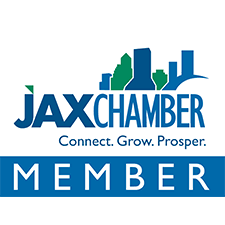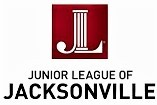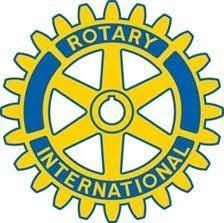## Thank you for your support!

 table div table+table+table+table+table+table+table+table+table+table+table+table+table+table+table+table+table+table+table+table+table+table+table+table+table+table+table+table+table+table+table+table+table+table+table+table+table+table+table+table+table+table+table+table+table+table div table{width:100%;padding:0}table div table+table+table+table+table+table+table+table+table+table+table+table+table+table+table+table+table+table+table+table+table+table+table+table+table+table+table+table+table+table+table+table+table+table+table+table+table+table+table+table+table+table+table+table+table+table div table img{width:96.23%;padding:0;float:none}table div table+table+table+table+table+table+table+table+table+table+table+table+table+table+table+table+table+table+table+table+table+table+table+table+table+table+table+table+table+table+table+table+table+table+table+table+table+table+table+table+table+table+table+table+table+table div table td{width:100%;padding:0 1.88% 18px}/* styles */## Staff:

Alan Bliss, Ph.D. , Executive Director | Mitch Hemann, Archivist | Susan Prattos, Administrator | Imani Phillips, Archives & Office Assistant | Sherrard Ceglia, Archives Assistant | Anna Verney, Archives Assistant | David Woodard, Facilities Manager